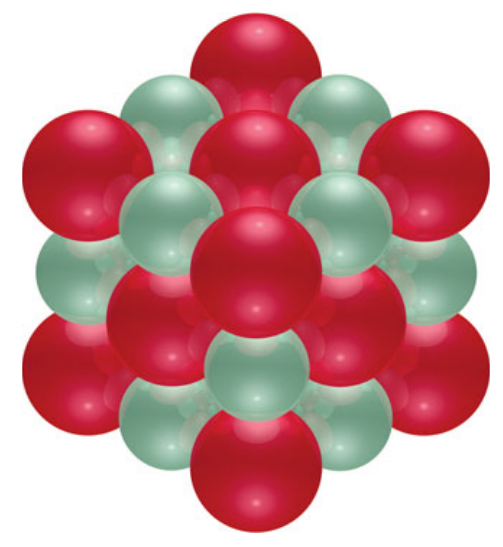×
Get Full Access to Chemistry: The Central Science - 12 Edition - Chapter 7 - Problem 92ae
Get Full Access to Chemistry: The Central Science - 12 Edition - Chapter 7 - Problem 92ae

×

# The ionic substance strontium oxide, SrO, forms from theISBN: 9780321696724 27

## Solution for problem 92AE Chapter 7

Chemistry: The Central Science | 12th Edition

• Textbook Solutions
• 2901 Step-by-step solutions solved by professors and subject experts
• Get 24/7 help from StudySoup virtual teaching assistantsChemistry: The Central Science | 12th Edition

4 5 1 248 Reviews
10
2
Problem 92AE

The ionic substance strontium oxide, SrO, forms from the reaction of strontium metal with molecular oxygen. The arrangement of the ions in solid SrO is analogous to that in solid NaCl (Figure 2.21):(a) Write a balanced equation for the formation of SrO(s) from its elements. (b) Based on the ionic radii in Figure 7.7, predict the length of the side of the cube in the figure (the distance from the center of an atom at one corner to the center of an atom at a neighboring corner). (c) The density of SrO is 5.10 g/ cm3. Given your answer to part (b), how many formula units of SrO are contained in the cube shown here?

Step-by-Step Solution:
Step 1 of 3

January 23 rd Atoms and molecules adopt shapes that maximize the distance between valence electron pairs. Ex: Methane (CH ) 4 - For 4 electron pairs, maximal distance occurs as a tetrahedral shape. - All bond angles in this shape are 109.5 degrees. But about the orbitals involved in bonding We use planes to show how electrons move around. However, if electrons bonded strictly to these orbitals, it would not result in a tetrahedral shape. Therefore, the orbitals are combined, or hybridized. Formulas and Isomerism There

Step 2 of 3

Step 3 of 3

##### ISBN: 9780321696724

Chemistry: The Central Science was written by and is associated to the ISBN: 9780321696724. Since the solution to 92AE from 7 chapter was answered, more than 418 students have viewed the full step-by-step answer. This full solution covers the following key subjects: sro, figure, ionic, solid, atom. This expansive textbook survival guide covers 49 chapters, and 5471 solutions. This textbook survival guide was created for the textbook: Chemistry: The Central Science, edition: 12. The full step-by-step solution to problem: 92AE from chapter: 7 was answered by , our top Chemistry solution expert on 04/03/17, 07:58AM. The answer to “?The ionic substance strontium oxide, SrO, forms from the reaction of strontium metal with molecular oxygen. The arrangement of the ions in solid SrO is analogous to that in solid NaCl (Figure 2.21): (a) Write a balanced equation for the formation of SrO(s) from its elements. (b) Based on the ionic radii in Figure 7.7, predict the length of the side of the cube in the figure (the distance from the center of an atom at one corner to the center of an atom at a neighboring corner). (c) The density of SrO is 5.10 g/ cm3. Given your answer to part (b), how many formula units of SrO are contained in the cube shown here?” is broken down into a number of easy to follow steps, and 116 words.

Unlock Textbook Solution